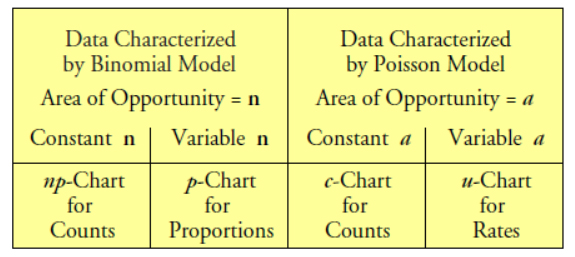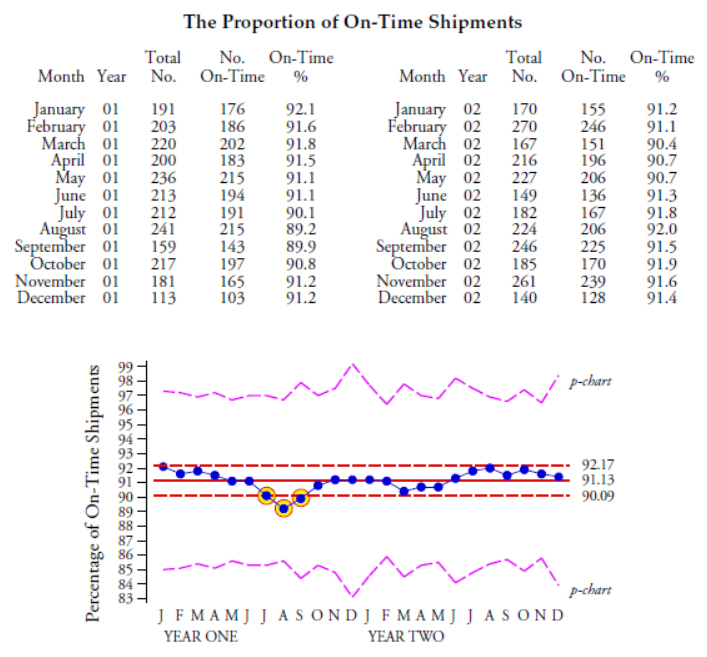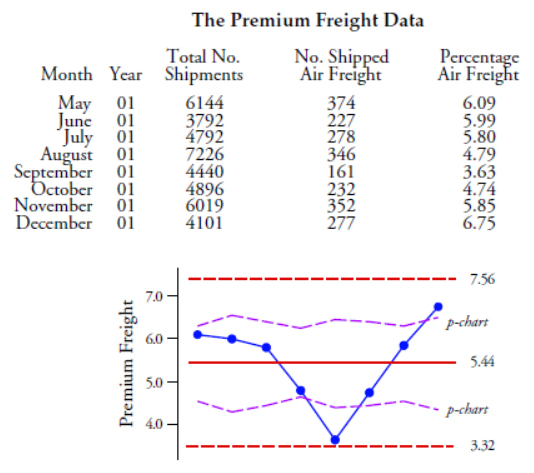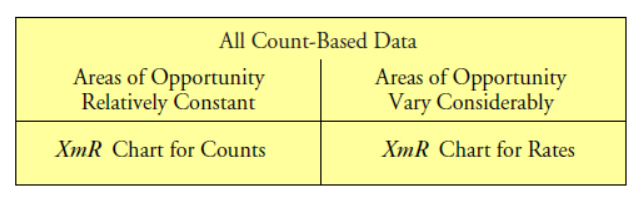#Quality Digest

Featured Product
This Week in Quality Digest Live
Statistics Features
W. Edwards Deming
More than 40 years later, has much changed? What do you think?
Donald J. Wheeler
How to know what the data are really telling you
Steve Moore
What math nerds do when they’re bored
Donald J. Wheeler
Not all count-based data will qualify
Jay Arthur—The KnowWare Man
Trend rules are helpful in service industries but you need to know which one to use
Statistics News
User friendly graphical user interface makes the R-based statistical engine easily accessible to anyone
Collect measurements, visual defect information, simple Go/No-Go situations from any online device
Good quality is adding an average of 11 percent to organizations’ revenue growth
Ability to subscribe with single-user minimum, floating license, and no long-term commitment
A guide for practitioners and managers
Gain visibility into real-time quality data to improve manufacturing process efficiency, quality, and profits
Tool for nonstatisticians automatically generates models that glean insights from complex data sets
Version 3.1 increases flexibility and ease of use with expanded data formatting features
Provides accurate visual representations of the plan-do-study-act cycleBio

Statistics

## So You Want to Use a p-Chart?

### Not all count-based data will qualify

Published: Monday, October 4, 2021 - 11:03

First, let it be known that all charts for count-based data are charts for individual values. Regardless of whether we are working with a count or a rate, we obtain one value per time period and want to plot a point every time we get a value. This need to plot the current data is why the specialty charts for count-based data were developed before a general approach for charting individual values was discovered. The question addressed in this column is when to use the specialty charts with your count-based data.

The first of these specialty charts, the p-chart, was created by Walter Shewhart in 1924. At that time the idea of using the two-point moving range to measure the dispersion of a set of individual values had not yet reached the professional literature. (John von Neumann would introduce the use of successive differences to the mathematical world in 1941, and W. J. Jennett would have the idea of an XmR chart in 1942.)

So the problem Shewhart faced was how to create a process behavior chart for individual values based on counts. While he could plot the data in a running record, and while he could use an average value as the central line for this running record, the obstacle was how to compute the limits. With the average and range chart Shewhart had used the within-subgroup variation, but this approach did not work with subgroups of size one. However, if the counts could be said to follow either a binomial probability model or a Poisson probability model, then the dispersion could be estimated from the average. So, lacking any alternative, Shewhart decided to use theoretical limits based on a probability model for his count-based data. And that is the origin of the specialty charts.Figure 1: Specialty charts for count-based data

Both probability models in figure 1 impose certain homogeneity conditions upon the data. Before a count of items can be said to be a binomial count each of the n items in any given time period must have the same probability p of possessing the attribute. Otherwise the sum of the n Bernoulli counts will not be binomial with parameters n and p. Before a count of events within some finite area of space or time or product, a, can be said to be a Poisson count, the events must logically be independent of each other and the events must be uniformly spread throughout the area of opportunity.

These models are fully specified by their mean value. This allows us to use the average to characterize both location and dispersion. Thus, p-charts, np-charts, c-charts, and u-charts all have limits that are based upon a theoretical relationship between the mean of a probability model and its dispersion. Hence, these specialty charts all use theoretical limits. If the counts can be reasonably modeled by either a binomial distribution or a Poisson distribution, then one of these specialty charts will provide appropriate limits for the data.

Over the years many textbooks and standards have forgotten that the assumption of a binomial model or a Poisson model is a prerequisite for the use of these specialty charts. This is a problem because there are many types of count-based data that cannot be characterized by either a binomial or a Poisson distribution. When such data are placed on a p-chart, np-chart, c-chart, or u-chart the theoretical limits obtained will be wrong.

So what are we to do? The problem with the theoretical limits lies in the assumption that we know the exact relationship between the central line and the three-sigma distance. The solution is to obtain a separate estimate of dispersion, which is what the XmR chart does: While the average will characterize the location and serve as the central line for the X chart, the average moving range will characterize the actual dispersion in the data and serve as the basis for computing the three-sigma distance for the X chart.

Thus, the major difference between the specialty charts and the XmR chart is the way in which the three-sigma distance is computed. The p-chart, np-chart, c-chart, and u-chart will have the same running record, and essentially the same central lines, as the X chart. But when it comes to computing the three-sigma limits the specialty charts use an assumed theoretical relationship to compute theoretical values while the XmR chart actually measures the variation present in the data and constructs empirical limits.

To compare the specialty charts with the XmR chart we shall use three examples. The first of these will use the data of figure 2. These values come from an accounting department which keeps track of how many of their monthly closings of departmental accounts are finished “on time.” The counts shown are the monthly numbers of closings, out of 35 closings, that are completed on time. The limits are based on years One and Two.Figure 2:The X chart and np-chart for the on-time closing data

Here both the np-chart and the X chart computations give essentially the same limits. (The upper limit value of 36.8 is not shown since it exceeds the maximum value of 35 on-time closings.) Here the two approaches are essentially identical because these counts seem to be appropriately modeled by a binomial distribution. If you are sophisticated enough to determine when this happens, then you will know when the np-chart will work and can use it successfully. On the other hand, if you do not know when a binomial model is appropriate, then you can still use an XmR chart. As may be seen here, when the np-chart would have worked, the empirical limits of the X chart will mimic the theoretical limits of the np-chart, and you will not have lost anything by using the XmR chart instead of the np-chart.

Our next example will use the on-time shipments for a plant. The data are shown in figure 3 along with both the X chart and the p-chart for these data. The limits are based on all 24 values.Figure 3: The X chart and p-chart for the on-time shipments

The X chart shows a process with three points at or below the lower limit. The variable-width p-chart limits are five times wider than the limits found using the moving ranges. No points fall outside these limits. This discrepancy between the two sets of limits is an indication that the data of figure 3 do not satisfy the binomial conditions. (Even if we had nothing but the binomial limits we would know these data are not binomial data due to the way the running record hugs the central line.)

Here the prob­ability of a shipment being on time is not the same for all of the ship­ments in any given month. This invalidates the binomial model and makes the theoretical p-chart limits incorrect. However, the empirical limits of the XmR chart, which do not depend upon the appropriateness of a particular probability model, are correct.

Our final comparison will use the data of figure 4. There we have the percentage of incoming shipments for one electronics assembly plant that were shipped using air freight. The limits are based on all 8 values. Two points fall outside the variable-width p-chart limits while no points fall outside the X chart limits.Figure 4: The X chart and p-chart for the premium freight data

Figure 4 is typical of what happens when the area of opportunity for a count of items gets excessively large. The binomial model requires that all of the items in any given time period will have the same chance of possessing the attribute being counted. Here this requirement is not satisfied. With thousands of shipments each month, the probability of a shipment being shipped by air is not the same for all of the shipments. Thus, the binomial model is inappropriate, and the theoretical p-chart limits which depend upon the binomial model are incorrect. The X chart limits, which here are twice as wide as the p-chart limits, properly characterize both the location and dispersion of these data and are the correct limits to use.

Thus, the difficulty with using a p-chart, np-chart, c-chart, or u-chart is the difficulty of determining whether the binomial or Poisson models are appropriate for the data. As seen in figures 3 and 4, if you overlook the prerequisites for a specialty chart you will risk making a serious mistake in practice. This is why you should avoid using the specialty charts if you do not know how to evaluate the appropriateness of these probability models.

In contrast to this use of theoretical models which may or may not be correct, the XmR chart provides us with empirical limits that are actually based upon the variation present in the data. This means that you can use an XmR chart with count-based data anytime you wish. Since the p-chart, the np-chart, the c-chart, and the u-chart are all special cases of the chart for individual values, the XmR chart will mimic these specialty charts when they are appropriate and will differ from them when they are wrong.

When the specialty charts with variable-width limits are appropriate, the fixed-width limits of an XmR chart will approximate limits based on the average-sized area of opportunity.Figure 5: An assumption-free approach for count-based data

Thus, when you are confident that your counts within each time period satisfy the requirements for either a binomial probability model or a Poisson probability model, you may safely use an np-chart, p-chart, c-chart, or u-chart. If the theory is appropriate, the theoretical limits will be correct. If you are wrong about the theoretical model for your count-based data, then the theoretical limits will be incorrect.

Of course, you can guarantee that you have appropriate limits for your count-based data by simply using the XmR chart to begin with. The empirical approach will always be right.

### Discuss### Donald J. Wheeler

Dr. Donald J. Wheeler is a Fellow of both the American Statistical Association and the American Society for Quality, and is the recipient of the 2010 Deming Medal. As the author of 25 books and hundreds of articles, he is one of the leading authorities on statistical process control and applied data analysis. Find out more about Dr. Wheeler’s books and on-line seminars at www.spcpress.com.

Dr. Wheeler welcomes your questions. You can contact him at djwheeler@spcpress.com

### XmR and Run Charts

Specialty charts are indeed more difficult to utilize and validate.  In the many years that I used charts, I found that people understand the XmR chart and Run Charts (median = centerline) much more readily that others.  The XmR chart has been descrivbed as the "Swiss Army Knife of Process Behavior Charts by Dr. Wheeler, and I have always found that to be the case.

### XmR vs c, np, p, u

I have found that varying limits in p/u charts cause people to start questioning why the limits vary and how the limits were calculated, which diverts attention from what the chart is telling us.

In the XmR chart, people often wonder why we need a Range chart, but the flat UCL/LCL are not cause for concern. The range chart is easy to explain (differences between points) and the X chart is the actual data.

In the Health Care Data Guide, Provost and Murray argue that p and u charts are more sensitive to special causes, but in my own experience this is more an exception than a rule.

So, as Donald has pointed out before, the XmR chart is the Swiss Army Knife of control charts. It works well and is easy to use. Best of all, people only have to understand one type of chart which makes it easier to train leaders and managers who have never seen an XmR chart before.

Any SPC software will calculate and display these charts effortlessly. Start using the XmR chart. You'll be surprised by how versatile it is.

### CUSUM EWMA

Is XmR chart better than a CUSUM or a EWMA chart?

CUSUM and EWMA are both slower to detect a change than is the XmR chart.

I have written complete chapters on each of these techniques in my Advanced Topics in SPC textbook.